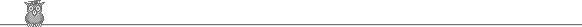### 23rd USAMO 1994Problem 3

The hexagon ABCDEF has the following properties: (1) its vertices lie on a circle; (2) AB = CD = EF; and (3) the diagonals AD, BE, CF meet at a point. Let X be the intersection of AD and CE. Show that CX/XE = (AC/CE)2.

Solution

Let the diagonals AD, BE, CF meet at Y. We show first that the triangles AEC, YED are similar. ∠ACE = ∠ADE (ACDE circle) = YDE (same angle). ∠AEB = ∠CED (AB = CD), so ∠AEB + ∠BEC = ∠CED + ∠BEC or ∠AEC = ∠YED. So AEC and YED are similar, so AC/CE = YD/DE.

We next show that AEC and CDY are similar. ∠AEC = ∠ADC (circle) = ∠CDY (same angle). ∠EAC = ∠EAD + ∠DAC = ∠ECD (circle) + ∠ECF (EF = CD) = ∠DCY. So AEC and CDY are similar. So AC/CE = CY/YD.

Hence (AC/CE)2 = CY/DE. Finally we show that CXY and EXD are similar. That is almost obvious because CF is parallel to DE since CD = EF. Hence CY/DE = CX/XE, which gives the required result.